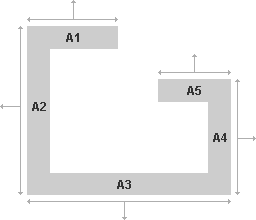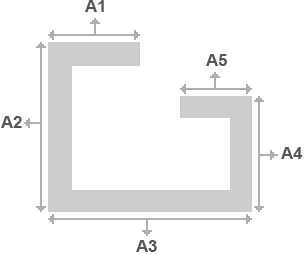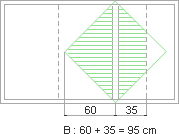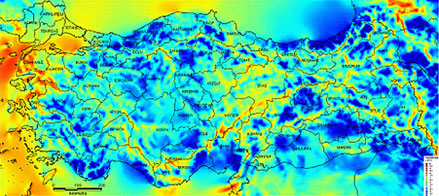## REINFORCEMENT PROFILE INERTIA VALUE CALCULATION#### RESULT

Inertia value on X axis lx

Inertia value on Y axis ly

Note: lx or ly is used according to the position of the reinforcement in the profile and accordingly to the direction of the pressure applied by the wind onto the reinforcement profile.

## WINDOW THERMAL CONDUCTIVITY COEFFICIENT CALCULATION

Width of glass
Height of glass

#### RESULT

Thermal Conductivity Coefficient

Note: Calculations are made in compliance with TS EN ISO 100077-1.

## WINDOW NOISE INSULATION VALUE CALCULATION

#### RESULT

Rw (dB)

C

Ctr

Note: The calculation method has been prepared in accordance with TS EN 14351-1 Appendix B.

## WINDOW WIND LOAD RESISTENCE CALCULATIONCalculation of the distance the wind load is effective on the window
"In the calculation of the B value, a 45 degree line is drawn from the two corners of the long side of the vane, if the distance of the intersection point of these two lines to the starting corner of the line is bigger than half of the vane length, half of the vane length, if equal or smaller than, the distance of the intersection point is taken. The calculations are made by being based upon the longest and the weakest side. The inertia values of the other sides will be smaller. If demanded, the calculations for each point can be made individually."

#### RESULT

Efficiency of the profile inertia

Deflection Amount AllowedAnual Wind Velocity Distribution (m/s) - 30 m

10 7,5 5 9,5 7 4,5 9 6,5 4 8,5 6 3,5 8 5,5 3

#### RESULT

Wind Velocity (m/s)

Wind Velocity (N/cm2)

Note: The calculation method has been prepared in accordance with TS EN 14351-1 Appendix B.